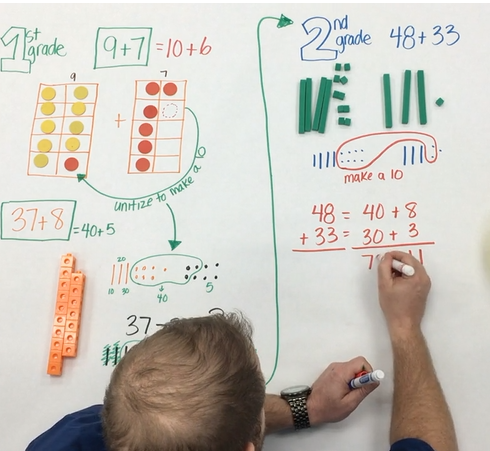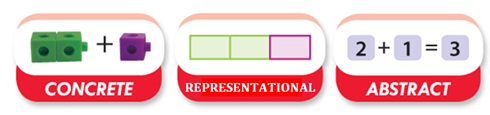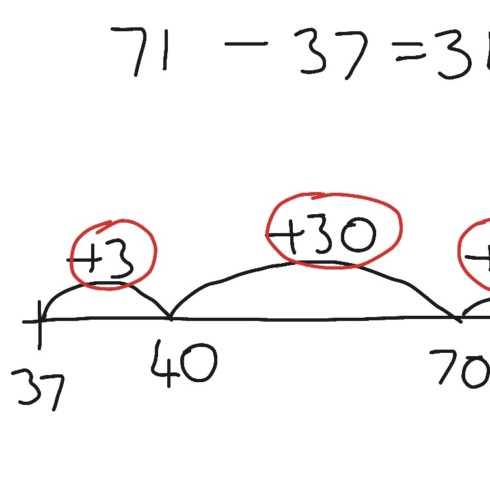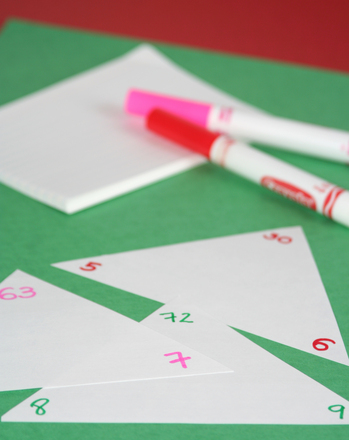## Archives

https://youtu.be/bfB0F5elm58 This video shows how Triangle Fact cards can be used to build quick recall with addition and related subtraction facts. These cards take the place of traditional flash cards, and help students see fact families and related facts which…It is the end of the school year. You've spent time and energy teaching various math concepts so that your students have a conceptual understanding of content. Students in your class have explored foundations of operations using concrete models and…According to MAFS.K.OA.1.1, students in kindergarten are required to, "Represent addition and subtraction with objects, fingers, mental images, drawings, sounds (e.g., claps), acting out situations, verbal explanations, expressions, or equations." They are also required to, "Solve addition and subtraction word…Do you have students in third, fourth, or fifth grade that are struggling with subtraction? Chances are, they missed the concrete phase of modeling subtraction with regrouping. This skill is extremely valuable as per the Mathematics Florida Standards, students in…The Progression of Addition and Subtraction is a wildly discussed topic in elementary schools. It is important that we do not rush students' understanding of the operations. Learning should be anchored in the foundational understanding of joining and separating situations,…In 4th grade students also use rounding to estimate sums and differences. This strategy is used to make sure that their estimation is reasonable. They used this strategy in 3rd grade. Below is some examples of estimation that students learned…Here are the Top 5 things to consider when teaching 3rd grade students about adding and subtracting multi-digit numbers with place value understanding: 1. Student’s prior knowledge from 2nd grade and how it relates to 3rd grade standards Students in…What is the Concrete-Representational-Abstract (CRA) instructional approach? CRA is a gradual systematic approach to teaching mathematics. Each stage builds on to the previous stage and therefore must be taught in sequence. This three step approach has been found to be…